﻿ Delaying of Exponential Solution When Using Integral Factor Analysis Method to Solve Differential EquationsPublications are Open
Access in this journal
Article Versions
Export Article
• Normal Style
• MLA Style
• APA Style
• Chicago Style
Research Article
Open Access Peer-reviewed

### Delaying of Exponential Solution When Using Integral Factor Analysis Method to Solve Differential Equations

Kajisa T.
Turkish Journal of Analysis and Number Theory. 2021, 9(1), 17-21. DOI: 10.12691/tjant-9-1-3
Received August 03, 2021; Revised September 05, 2021; Accepted September 14, 2021

### Abstract

It was confirmed that the results given by the integral factor method showed the delaying of response in the numerical experiments using the advection-diffusion equation. However, the exponential solutions given by the integral factor method were not very smooth compared to the analytically correct solution. On the other hand, a delay in the exponential solution was clearly found for an increasing time increment. Therefore it is important to make the time increment shorter step by step, to check the delaying when applying this integral factor method. It would be expected that the exponential solution given by the integral factor analysis method shown here would have the same expression. That would mean that this method had great potential and could be widely used.

### 1. Introduction

For engineers, for example, a basic ability to solve partial differential equations is often needed. Some of the equations include terms of the first and second orders of the differential of the distance, and some include a diffusion term, as the advection–diffusion equation that follows.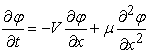(1)

In which,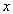is distance,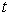is time,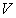and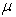are the constant.

The right-hand side of equation (1) is often converted to a finite differential expression for distance. In such a case, equation (1) will be an ordinal differential equation to which, sometimes, the integral factor method can be applied.

Kitagawa and Muramaki 1 show that, about the solution given by the integral factor method, there is no cut-off error due to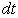and no numerical instability caused by an increase in the discrete step length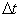for time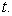The solution from the integral factor method is expressed as an exponential function. It is therefore the same as the solution using the method called the exponential finite difference method (Inan and Bahadir 2). The “explicit exponential finite difference method” was originally developed by Bhattacharya 3. It seems that there are two ways to develop the original method. The first is to make the basic scheme of the equation implicit 2, and the other is to make the exponential function have a larger order, following the exponential Taylor method (Koskela and Ostermann 4), or to add the optional term, (Ayinde and Ibijola 5).

On the other hand, the potential for the application of the integral factor method is so great that the equation is needless to include a diffusive term (see Figure 1). In engineering, the exponential solution given by applying the integral factor method looks very helpful because there is no rigid condition on getting a stabilized solution. This had been used in a real application in soil science, for example by Kajisa and Ermolin 1. However the property of the errors of this method still does not seem clear. Therefore, engineers need to know the kind of incorrect solution that can appear in this stabilized solution. For discussion, in this paper, the integral factor method is applied to equation (1).

### 2. Numerical Experiments

2.1. Finite Difference Method (Method A)

As the explicit method to solve equation (1), the following transformed equation is often used.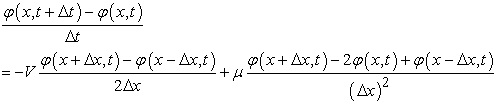(2-a)

The solution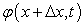is given analytically as follows: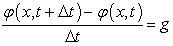(3-a)

Because in this paper we are interested in the explicit method,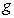includes no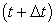term, as follows: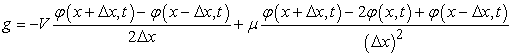(4-a)

The solution is expressed as follows from (3-a):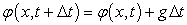(5-a)

From Savović and Djordjevich 6, the next two equations are used as the conditions for the solution.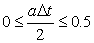(6-a)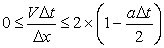(7-a)

In which,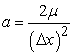(8)

The method in which equations (2-a)-(7-a) are used is hereafter called method A.

• Figure 1. An example of the process followed to get a stabilized exponential solution by the integral factor method
2.2. Integral Factor Method (Method B)

To make the correctness up,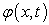in equation (4-a) is changed to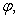which includes the continuous time fromto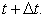Although only the summarized ordinary differential equation is shown in the reference, we accept it as follows.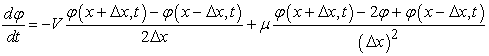(2-b)

Therefore,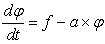(3-b)

in which, not only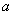but also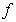do not include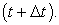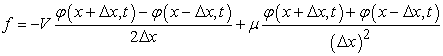(4-b)

The parameterdo not includedTherefore, it is defined as a constant in this paper. Thethat appears in the right-hand side in equation (4-a) is treated as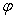in equation (1), in whichchanges fromtocontinuously.

The solutionis given analytically as follows, by integrating (3-b) for time fromto.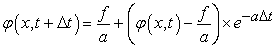(5-b)

The aim of this paper is to discuss the properties of using equation (5-b), as compared with equation (5-a).

In 7, the method using (3-b) is called the “integral factor method”. From 7, since the integral factor method solves the equation analytically, the cut-off error due todoes not occur if there is no numerical instability caused by the increasing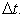Although in 1 we proposed the next equation (9) as the condition for the use of (5-b), only (6-a) is used in this paper, because it is duplicated.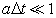(9)

The method in which equations (2-b)-(5-a) are used is called method B hereafter.

2.3. The Analytical Solutions (Method C)

Because the correct analytical solutions are different according to the initial or boundary conditions, the solutions were shown and discussed in a style of the step response and the impulse response as following the system engineering. The analytical method here is hereafter called method C.

2.3.1. The Step Response

As the conditions, the next three equations are used for the step response.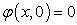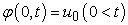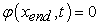For such conditions, the analytical solution is as follows 6: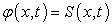(10)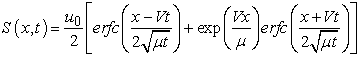(11)

2.3.2. The Impulse Response

As the conditions, the next three equations are used for the impulse response.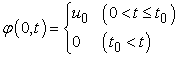For such conditions, the analytical solution is as follows: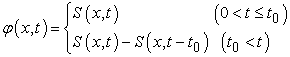(12)
2.4. The Simulations

Hereafter, the suffixes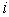and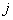are used to express the distanceand time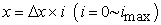(13)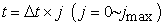(14)

For the three predicted methods, the three simulations that follow (case 1, case 2, and case 3) are carried out for discussion purposes. The properties of these simulations are shown in Table 1. In any case in Table 1, the conditions shown in (6-a) and (7-a) are not strongly satisfied for the purposes of this paper.

For the experiment, as an example,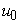is 1,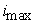for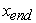is 200. In Table 1, time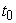is defined as the time used in the impulse response, with 10 as an example.

Becauseis 0.25, 0.50, 0.75,foris 40, 20, and 14 for case 1, 2, and 3, respectively. Time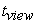defined as the time for showing the results is 36. Therefore,foris 144, 72, and 48 for case 1, 2, and 3, respectively.

### 3. Results

• Figure 2. Step response by method A (tview is 36)

The solutions are given by the three methods A, B, and C for cases 1, 2, and 3. The results for the step response are shown in Figure 2 and Figure 3. After that, the results for the impulse response are shown in Figure 4 and Figure 5.

In Figure 2, for the finite difference method (method A), the numerical instability is shown only for case 3, for which the conditions of equation (6-a) and (7-a) are not satisfied.

In Figure 3, for the integral factor method (method B), no numerical instability is shown, as predicted. The delay made by method B compared with the correct solution by method C gets larger for the cases from 1 to 3.

In Figure 4, for the finite difference method (method A), numerical instability is shown only for case 3. In detail, the peak of case A was larger than the peak given by the correct method C in case 2.

In Figure 5, for the integral factor method (method B), no numerical instability is shown, as predicted. The delaying made by method B compared with the correct solution by method C gets larger for the cases from 1 to 3. In detail, the peak is smaller than the correct solution by the method C in case 2.

• Figure 3. Step response in method B and C (tview is 36)
• Figure 4. Impulse response by method A (tview is 36)
• Figure 5. Impulse response by method B and C (tview is 36)

### 4. Discussion

Before looking at the results, it would have been presumed that the solutions would show a moving average by method B, because it is known to show no instability. However, the six graphs of results by method B shown in Figure 2 and Figure 5 are not very smooth compared with the correct solution by method C. We note a delay in Figure 3 and Figure 5, and the smoothing is not easy to see. The peak value would not have the large error.

By both method B and C, the result for case 1 seems better than that for case 2. Therefore it is important for engineers to test how their results change by settingto be smaller and smaller after getting the first solution. The instability often stops the simulation system. Therefore, Method B that show no instability, would be helpful for the engineers. The delaying will be almost the only weak point for the engineering to take care.

### 5. Summary

1. It was confirmed that the results given by the integral factor method showed the delaying of response in the numerical experiments using the advection-diffusion equation.

2. For applying this method, the basic equation model are needless to have the exact diffusion term. That would mean that this method have the ability to be widely used to get the smoothed solution.

3. For this kind of exponential solution, there will be no instability to stop any numerical simulation. Therefore, it is recommended that the delaying to be checked by making the time increment shorter and shorter.

### Acknowledgements

The term “integral factor method” is used to mean “exponential Taylor method (of the first order)” in this paper. Additionally, “the exponential function method” and the “exponential finite difference method” are almost the same here. Some of these terms seem to be used for engineering studies and others for mathematics studies. They are not distinguished here in detail, because we have mentioned the explicit first order problem.

### References

  Kajisa T., Ermolin Y., The stabilized answer of diffusion equation for unsteady 3-D unsaturated subsurface flow analysis, December 2000, International Agricultural Engineering Conference, Asian Institute of Technology, Thailand, 2000. https://www.researchgate.net/publication/343787565. In article  Inan B., A.R. Bahadir, Numerical solution of the one-dimensional Burgers' equation: Implicit and fully implicit exponential finite difference methods, Pramana, 2013. https://link.springer.com/article/10.1007/s12043-013-0599-z. In article View Article  Bhattacharya M.C., Int. J. Numer. Methods Eng. 21, 239 (1985). In article View Article  Koskela A. and Ostermann A., Exponential Taylor methods: Analysis and implementation, A Ostermann - Computers & Mathematics with Applications, 65, 487-499, 2013. In article View Article  Ayinde S.O., Ibijola E.A., A new Numerical Method for Solving First Order Differential Equtions, American journal of Applied Mathematics and Statistics, vol. 3, no. 4, 2015: 156-160. In article  Savović S., Djordjevich A., Explicit finite difference solution of for contaminant transport problems with constant and oscillating boundary conditions, Thermal Science, May 2020. In article View Article  Kitagawa M., Murakami Y., Stable and highly accurate numerical solution of nonlinear wave equations, New progress in research on nonlinear wave phenomena, Research Institute for Mathematical Sciences, 1800: 226-235, 2012 (in Japanese). In articleThis work is licensed under a Creative Commons Attribution 4.0 International License. To view a copy of this license, visit http://creativecommons.org/licenses/by/4.0/